# 3 Ways of Counting Happy Triangles

I love counting problems and here is one of my favourites. How many triangles can be made using the vertices and edges of the diagram below such that it is orientated the same way as ABC?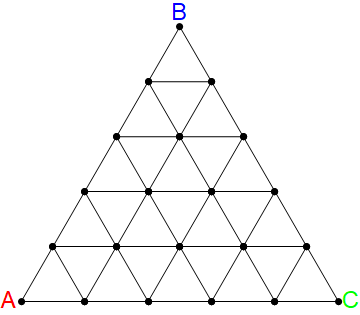If you counted exactly 35, that's right! Counting them all by hand can get a bit messy so maybe you found an easier way. Here are a couple of my favourites that we can summarise non-rigorously now.

# Two ways of counting

1. Count the number of valid triangles of length $l$ for each $l \in \{1,2,...,n\}$. We find that for each given $l$ there are $1 + 2 + ... + n+1-l =$ $n+2-l \choose 2$. This gives the sum of all the triangles to be $\sum_{l=1}^{l=n}$$n+2-l \choose 2$.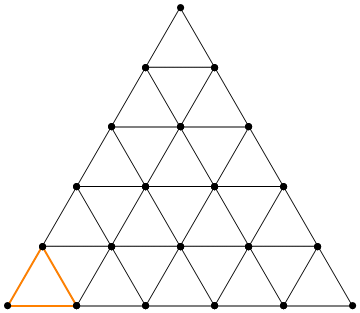1. Another way we could try to count the triangles is like so. Fix a single vertex that might be the top of some valid triangle and ask how many valid triangles have that as a "top vertex". For a vertex on the $h^{th}$ horizontal line from the lowest horizontal line, there are $h$ valid triangles that use it as a "top vertex".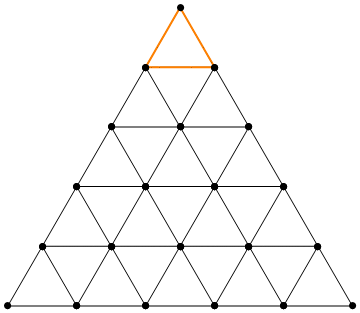This means that the number of valid triangles that have a vertex on the the $h^{th}$ horizontal line is given by the number of vertices in that line, multiplied the number of valid triangles that use a given vertex as its top. This gives $(n+1-h)h$ triangles that have a vertex on the the $h^{th}$ horizontal line. Now this gives the formula for the number of valid triangles $\sum_{h=1}^{h=n}(n+1-h)h$.

# What just happened?

These counting arguments gave us two different formulas for how to count the triangles which were connected to two different ways of counting the triangles. Since they are counting the same thing this gives us a cute combinatoric identity that $\sum_{h=1}^{h=n}(n+1-h)h$ = $\sum_{l=1}^{l=n}$$n+2-l \choose 2$.

With a little effort and algebraic manipulation, we can use some more identities to prove that both of these formulas are also equal to the expression $n+2 \choose 3$. $\color{#BBBBBB}\text{Can you prove this?}$ Before we started with a method of counting things and derived a mathematical formula. In this case, we are given a formula for counting the number of triangles, $n+2 \choose 3$, but we did not get it explicitly from counting triangles... spooky!

So the question is, does there exist a way of counting the triangles connected to this formula? Is there a way of having n+2 objects such that when we choose any 3 of them it determines a unique valid triangle? I strongly encourage you to try to think about this before reading on.

# A 3rd Way of Counting the Triangles

We'll show a way of counting the triangles inspired by the formula $n+2 \choose 3$. We won't go into the rigour of why it works but I think that's a fun exercise in itself! First of all, we are going to need to decide on some $n+2$ objects for which choosing 3 of them will determine a valid triangle. For this, we will use the vertices of the bottom row along with some newly created vertex to the left of these. We will label these vertices with the numbers 1,2,...,n+2 starting from left to right as shown below. We will also assume that all the edges between adjacent vertices in our diagram are of unit length.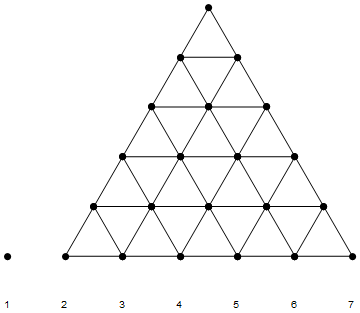Now we will select 3 of these labelled vertices and determine a triangle from it. We will call the vertices chosen L, M, R for the left most, the middle one and the right most labelled vertex selected. We let the values or L, M, R be their labels to make it easier to explain ideas.

We will use the label of L to determine the length of the triangle we will determine. We will use M and R to determine the position of the valid triangle of length L we are interested in. Here's how it works in detail.

First, we define the point H of a valid triangle to be the point, on the edge between "the bottom right vertex" of the valid triangle and "the top vertex", that is distant 1 from the bottom right vertex. Here is an animation to try make this clear. The orange triangles are valid triangles and the highlighted blue vertex is its corresponding H point.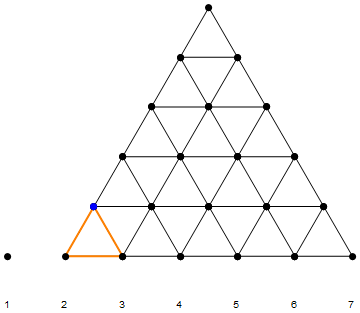Ok, now we have some terminology we can describe the algorithm for corresponding a valid triangle to 3 of our n+2 labelled points.

1. Select the distinct vertices L, M and R in our n+2 labelled vertices.
1. This corresponds to the triangle of length L that has its H vertex at the point that is the top vertex of the valid triangle that has M and R as vertices.

Let's see what this looks like!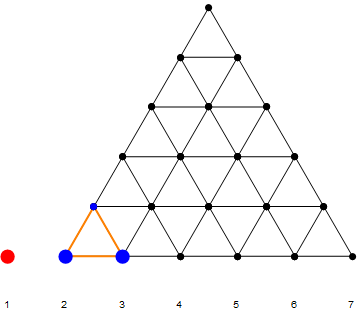# Checking this works

Ok, so now we should prove that this actually works! So what do we need to do? We need to check that this process is reversible and works for all n. I am feeling extremely lazy and will not fill in the details here now but a lot of it can be seen by using induction.

# Thoughts & Questions

• These three formulas seem fundamentally different in some way how might we formalise this idea?
• We could consider these formulas and the algorithms that correspond to them and think about the kind of "For Loops" they have and when we might be able to get rid of a given for loop. When is this possible? If a nice formula for counting some objects exists, when can we find an algorithm to count the objects in a corresponding way? For example, the number of squares that can be made in an $n \times n$ grid can be given as $n^2 \choose 2$$/3!$= $n+1 \choose 3$$\frac{n}{2}$ - are there any nice ways of finding algorithms that correspond to these formulas?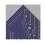Note by Roberto Nicolaides
4 years ago

This discussion board is a place to discuss our Daily Challenges and the math and science related to those challenges. Explanations are more than just a solution — they should explain the steps and thinking strategies that you used to obtain the solution. Comments should further the discussion of math and science.

When posting on Brilliant:

• Use the emojis to react to an explanation, whether you're congratulating a job well done , or just really confused .
• Ask specific questions about the challenge or the steps in somebody's explanation. Well-posed questions can add a lot to the discussion, but posting "I don't understand!" doesn't help anyone.
• Try to contribute something new to the discussion, whether it is an extension, generalization or other idea related to the challenge.

MarkdownAppears as
*italics* or _italics_ italics
**bold** or __bold__ bold
- bulleted- list
• bulleted
• list
1. numbered2. list
1. numbered
2. list
Note: you must add a full line of space before and after lists for them to show up correctly
paragraph 1paragraph 2

paragraph 1

paragraph 2

[example link](https://brilliant.org)example link
> This is a quote
This is a quote
    # I indented these lines
# 4 spaces, and now they show
# up as a code block.

print "hello world"
# I indented these lines
# 4 spaces, and now they show
# up as a code block.

print "hello world"
MathAppears as
Remember to wrap math in $$ ... $$ or $ ... $ to ensure proper formatting.
2 \times 3 $2 \times 3$
2^{34} $2^{34}$
a_{i-1} $a_{i-1}$
\frac{2}{3} $\frac{2}{3}$
\sqrt{2} $\sqrt{2}$
\sum_{i=1}^3 $\sum_{i=1}^3$
\sin \theta $\sin \theta$
\boxed{123} $\boxed{123}$